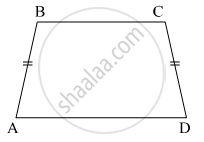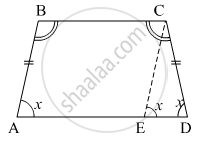Advertisement Remove all ads

# In □ Abcd , Side Bc < Side Ad (Figure 5.32) Side Bc || Side Ad and If Side Ba ≅ Side Cd Then Prove that ∠ Abc ≅ ∠ Dcb. - Geometry

Sum

In squareABCD , side BC < side AD in following figure .
side BC || side AD and if side BA ≅ side CD then prove that ∠ ABC ≅ ∠ DCB.Advertisement Remove all ads

#### SolutionConstruction: Draw a line CE || AB
CE || AB          (By construction)
AE || BC          (Give)
So, ABCE is a parallelogram.
Since, AB || CE
⇒ ∠BAE = ∠CED = ∠x
(Corresponding angles)         .....(1)
Now, BA ≅ CE                           (ABCE is a parallelogram)
and BA ≅ CD                              (Given)
So, CE ≅ CD
⇒ ∠CED = ∠CDE = ∠x
(Isosceles triangle)               .....(2)
From (1) and (2) we have
∠BAE = ∠CDE = ∠x      .....(3)
∠CEA = 180º − ∠CED = 180º − ∠x
In a parallelogram, the opposite angles are equal.
So, ∠ABC = ∠CEA = 180º − ∠x     .....(4)
Similarly, ∠BAE = ∠BCE = ∠x
In ∆CED,

∠ECD + ∠CED + ∠EDC = 180°

⇒ ∠ECD + x + x = 180°

⇒ ∠ECD = 180° - 2x

Now ∠DCB = ∠BCE + ∠ECD
= x + 180º − 2x
= 180º -x
Thus, ∠ ABC ≅ ∠DCB

Is there an error in this question or solution?
Advertisement Remove all ads

#### APPEARS IN

Balbharati Mathematics 2 Geometry 9th Standard Maharashtra State Board
Chapter 5 Quadrilaterals
Practice Set 5.4 | Q 3 | Page 71
Advertisement Remove all ads
Advertisement Remove all ads
Share
Notifications

View all notifications

Forgot password?